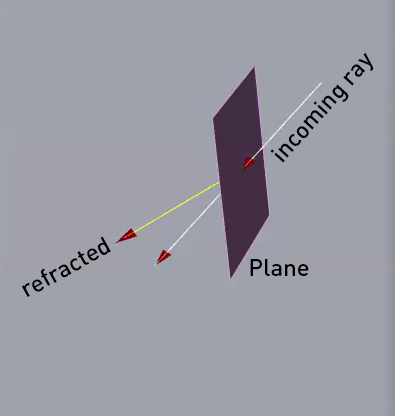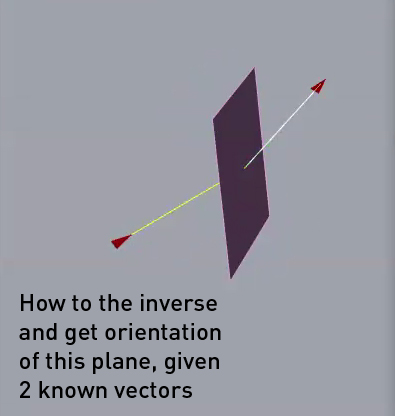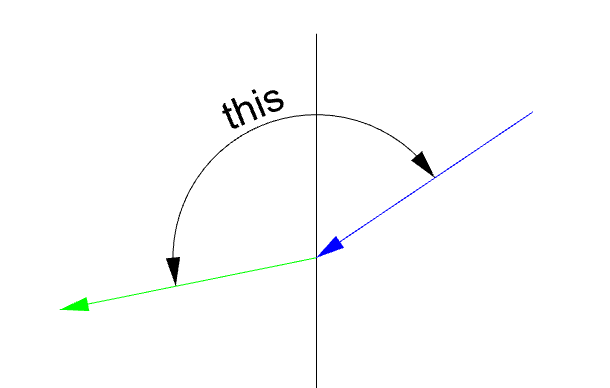# Howto calculate orientation of plane given 2 vectors (inverse Snells Law)

Hi, I am struggling to get my hand around the following. Using Snell’s Law it’s easy to the refraction of a light ray if it passes across the boundary separating two media. In my example that boundary is air and simple Plane with a certain IOR. For this I am using the component from Mosquito plugin: Mosquito - Synthetic SpacesHowever in my case I need to the exect opposite, and calculate the orientation of this Plane, given that I know both vectors (the incoming and outcoming one).It seems super simple, yet I fail to get there
I attach this Refraction script, of first image
Refract_Inverse.gh (15.1 KB)

this is a 10-seconds-of-focus approachif you have this angle between the two vectors:then there should be just one configuration (with given indexes of refraction n1, n2) that produces exactly that angle:

given the formula n1 * sin(theta1) = n2 * sin(theta2) and that you know n1 and n2, you might just populate theta1 in the range (0,Pi/2] to find the theta2, and so to find this for each theta_1 in the list and graph it down:

Refract_Inverse_Re.gh (25.7 KB)

@inno , You just saved my weekendAwesome. Figured it would be something along these lines, but I had a hard time seeing it work for a 3d plane.yeahjust for the fun of it, by representing the curve in 3D with (XYZ) as (theta_1, theta_2, in-between-angle) you can easily find all the values like this:

Refract_Inverse_Re_Re.gh (25.3 KB)

Hi @inno , I thought I understand where you were going with the suggested script, but I fail to exactly translate this for my situation. Would you mind extending this script where this oriented plane is placed, given just the 2 incident and refracted rays (and the 2 IOR’s) (as depicted in my [second image] of the first post (https://global.discourse-cdn.com/mcneel/uploads/default/original/4X/3/7/e/37ed17e004860c1b89e735d653a91d18f3b29580.jpeg)). So If I change the direction of either or both incident and refracted rays, the oriented plane adjust accordingly

For now I’ll stick with the workaround using Galapagos to find the best fitness for the orientation axis of this plane (starting from a plane centered at the start of the incident ray).

Again, thanks for the helping out so far, and I will keep trying to find a solution in the mean while

I’m a bit running, this is a 2D representation of what happens in 3D (you can rebuild it or just Orient it)

it takes a polyline made of 3 points where 0-1 is first vector and 1-2 is the second:

calculates the angle between them and feeds that to the definition I posted earlier with the 3Dcurve in order to get back theta_1 and theta_2:

builds a plane P (white) based on the direction of the plane normal Pn rotated by theta_1 value (theta_1 and theta_2 are re-measured with the Angle Dimension Component just as final confirmation that all the numbers are right)

Refract_Inverse_Re_Re_Re.gh (34.1 KB)

Check this as well
It give you the plane used to calculate the reflection curve and the angle from the source point

Refract_Inverse.gh (58.0 KB)

1 Like